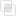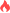c语言中缺省参数的两种类型

c语言 缺省参数2021-10-12 09:47:021017浏览 · 0收藏 · 0评论1、函数全缺省参数，函数在定义或者声明时，所有的形参都默认值。

#include <stdio.h>
//x,y为函数的形参，如果函数被调用时，没有设置x和y值，x值默认为100，y值默认为5
int sub(int x=100,int y=5)
{
return (x-y);
}
int main(void)
{
int a=20;
int b=10;
//变量a、b为sub函数的实参
printf("sub函数计算结果 = %d\n",sub(a, b));
//注意：我们并没有为sub函数设置实参，此时默认x=100 y=5
printf("sub函数计算结果 = %d\n",sub());
return 0;
}
/*

sub函数计算结果 = 10
sub函数计算结果 = 95
*/

2、函数半缺省参数，函数在定义或者声明时，只有一部分形参有默认值。

#include <stdio.h>
//x,y为函数的形参，如果函数被调用时，没有设置y值，y值默认为5

int sub(int x,int y=5)
{
return (x-y);
}
int main(void)
{
int a=20;
int b=10;
//变量a、b为sub函数的实参
printf("sub函数计算结果 = %d\n",sub(a, b));
//注意：我们并没有为sub函数第二个参数设置实参，此时默认y=5
printf("sub函数计算结果 = %d\n",sub(a));
return 0;
}
/*

sub函数计算结果 = 10
sub函数计算结果 = 15
*/c语言strcat_s函数是什么c语言strcat_s函数如何使用c语言strcat_s函数的原理c语言中如何计算数组长度c语言中的指针是什么c语言中数组访问越界如何理解c语言中如何防止数组下标越界c语言中函数的理解c语言中函数返回值的讨论c语言中函数参数类型的探究c语言中函数的声明和定义c语言野指针产生的原因c语言如何避免野指针产生c语言中常规函数和指针函数区别c语言中回调函数的使用js事件委托如何理解8228

js中hasOwnProperty的属性用法7373